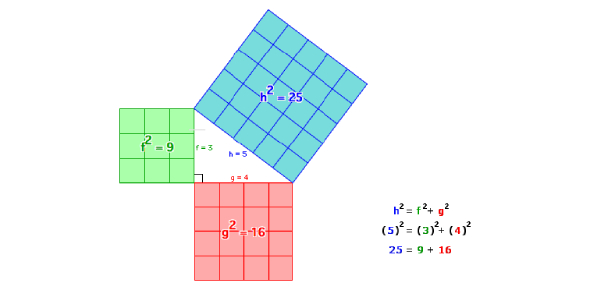How is the Pythagorean Theorem true for n dimensional solids? - ProProfs DiscussTopicsMoreProductsMore+ Ask Question

# How is the Pythagorean Theorem true for n-dimensional solids?

Asked by M. Jabrowsky, Last updated: Dec 18, 2019

###Request 0FollowShareAnswer AnonymouslyAnswer LaterCopy Link#### John F. connor

Traveler, Avid Reader, Free as a bird

John F. connor, Content Marketing executive, MA, Minsk,PolandSolid modeling refers to three-dimensional models. When referring to n-dimensional solids, that means that there are shapes, but it could different dimensions. It could be two or three dimensions. There are ways to apply the Pythagorean theorem to three-dimensional shapes if the shape has a right angle in its triangle.Just like a 2-dimensional shape, the student would need to know the two sides that have the right angle in between them. Then they could calculate the hypotenuse using the theorem of A squared plus B squared is equal to C squared. For a cube, a line can be drawn to make the right triangle. Then as long is one side is known, the theorem can be applied and the cube can be calculated.Search for Google imagesSelect a recommended image
Upload from your computerCancelSearch for Google imagesSelect a recommended image
Upload from your computerCancelSearch for Google imagesSelect a recommended image
Upload from your computerCancel